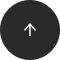Standard SQL

# How to Compute an Absolute Value in SQL

ABS

#### Problem:

You want to find the absolute value of a number.

#### Example:

You want to compute the absolute value (a.k.a. modulus) of each number in the column `numbers` from the table `data`.

numbers
-3.2
0
20

#### Solution:

```SELECT
ABS(numbers) AS absolute_values
FROM data;
```

The result is:

absolute_values
3.2
0
20

#### Discussion:

To compute the absolute value of a number, use the `ABS()` function. This function takes a number as an argument and returns its value without the minus sign if there is one. The returned value will always be non-negative – zero for argument 0, positive for any other argument. Note that the returned value will differ from the argument only if the argument is negative.

If, for some reason, you need to convert only the positive values to their negative equivalent and leave the other (i.e., zero and negative) values untouched, you can use a minus sign before the `ABS()` function.

```SELECT
-ABS(numbers) AS non_positive
FROM data;
```

The result will be:

non_positive
-3.2
0
-20

#### Recommended articles: